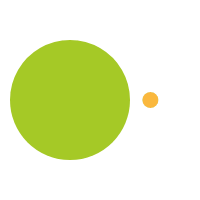May 18, 2016

# Three things to help prevent summer math loss.

## Repetition:

Have your student keep those math facts fresh in their mind by playing games that repeat the basic operations: adding, subtraction, multiplication and division.  Easiest way to do this is through math games that use cards, dice or spinners as they will give the child repetition without the use of stale worksheets.  They can roll dice, spin a spinner or draw cards to create randomly generated numbers that they can then add, subtract, multiply or divide.  Money is another way to get student to do repetitive math practice.  Have them add, subtract, multiply and divide monetary amounts.  This will help with algorithms and also a good introduction or reinforcement of decimals.

## Explanation thru explorations:

Provide your students with pattern blocks, tiles, centimeter cubes, graph paper, etc. and let them explore thing like area, perimeter, and volume.   Through this play students will get an understanding of the formulas for area, perimeter, rectangles, triangles, trapezoids, etc.   Have them work with rulers, yard sticks, measuring tape and discover inches, feet, centimeters, and millimeters.   They can understand things like even and odd numbers by making partners with objects.  If they learn through exploration then the information stays with them longer than if they are just given a random fact to memorize.

## Problem solving:

Play games like chess, checkers, Blokus, Mancala etc.  Games where they not only have to think of the first move but what their next move should be.   Get students to think strategically and deductively.  These games should be played repetitively so students can become better strategically and find success.  First time through any game students are just trying to figure out how to play, second time through they  are starting to think about the rules and it isn’t until the third time through that they start to think strategically and can start applying some deductive reasoning.

{% if context == "cart" or context == "checkout" %} {% endif %}